Next:Distance MeasuresUp:Relative Information and Inaccuracy

Properties of Information Measures

Some properties of the measures (2.1) and (2.2) are presented in this subsection. For simplicity, let us denote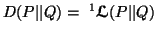and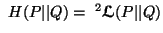.

Property 2.1. (Continuity).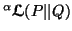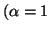and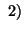are continuous functions of the pair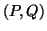.

Property 2.2. (Symmetry).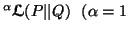andare symmetric functions of their arguments in pair, i.e., for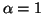and 2, we have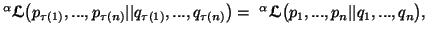where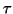is any permutation from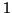to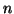.

Property 2.3. (Expansibility). Forand 2, we have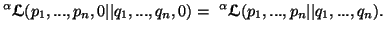Property 2.4. (Additivity). Forand 2, we have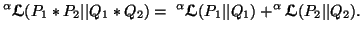where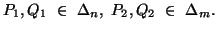Property 2.5. (Sum representation). We can write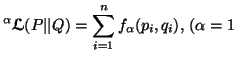andwhere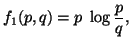and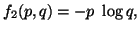for all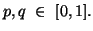Property 2.6. (Nonnegativity).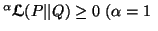and, with equality iff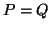forand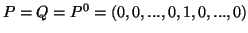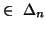for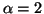.

Property 2.7. (Recursivity). Forand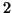, we have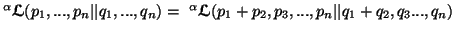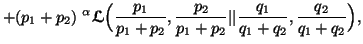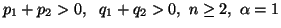and.

Property 2.8. (Strongly additive). For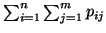=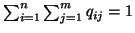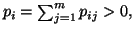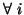,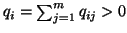, we have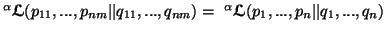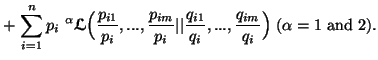Property 2.9. (Functional equation). Let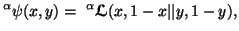then forand 2, we have
(i)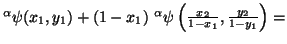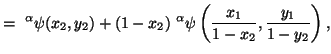for all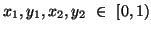with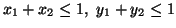.
(ii)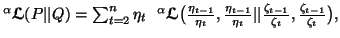where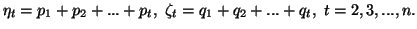Property 2.10. (Parallelogram identity). For any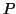,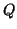,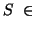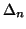, we have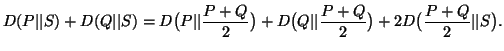Property 2.11. (Convexity). We have
(i)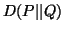is a convex function in a pair of distributions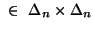.
(ii)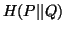is a convex function in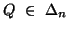.
Property 2.12. (Data processing inequality). Let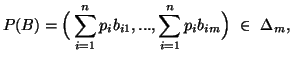and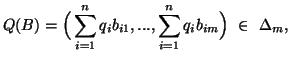be two probability distributions, where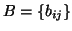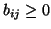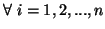;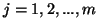is a stochastic matrix such that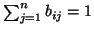. Then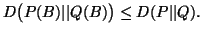Property 2.13. (Schur-convexity). We have
(i)is a Schur-convex function in the pair.
(ii)is a Schur-convex function in.
Property 2.14. (Inequality). We have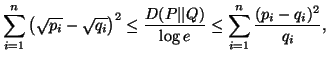with equality iff.

21-06-2001
Inder Jeet Taneja
Departamento de Matemática - UFSC
88.040-900 Florianópolis, SC - Brazil